Wednesday, 19 October 2011

Subtraction 1

Subtract the number at the top of the table from each of the numbers in the left column.
 Subtract 0 8 6 2 10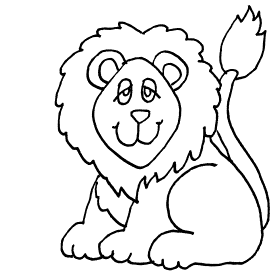Subtract 4 5 4 9 7
 Subtract 5 5 8 10 6
 Subtract 1 6 5 8 3
 Subtract 3 10 6 7 3

Division 1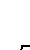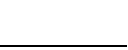7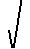196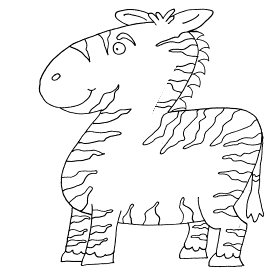254101802785470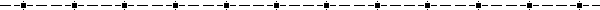Division 2

 15 ÷ 3 =        2 ÷ 2 =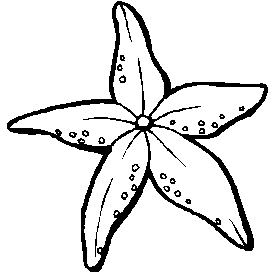30 ÷ 6 =        4 ÷ 4 =        24 ÷ 3 =        10 ÷ 1 = 3 ÷ 1 =        100 ÷ 10 =        21 ÷ 7 =        32 ÷ 4 =Multiplication 1

 9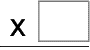72 660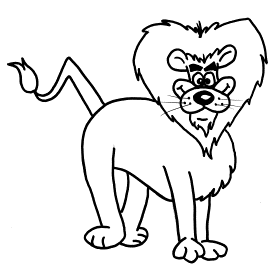856 99 864 515 816 535 428 525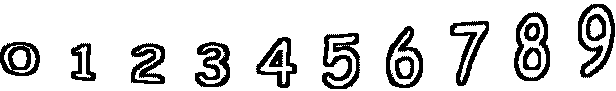Multiplication 2

One of these days I'm not going to have to work on the website at all anymore -- my children will do everything *laugh*.  Kaitlyn worked very hard on this little math crossword for a school project with disconcertingly little help from mom or dad.  You go girl!
Click on the image below to see it in its own window (close that window to return to this screen) OR
Right click and save image to your hard drive to print from your own  image software at your convenience.

Subtraction 2

Subtract the number at the top of the table from each of the numbers in the left column.
 Subtract 0 8 6 2 10Subtract 4 5 4 9 7
 Subtract 5 5 8 10 6
 Subtract 1 6 5 8 3
 Subtract 3 10 6 7 3

 12    + 4 19    + 7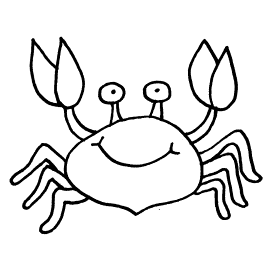12    + 4 11    + 1 18    + 2 17    + 0 11    + 6 10    + 6 10    + 2 14    + 31 6 + 7 1 2 + 5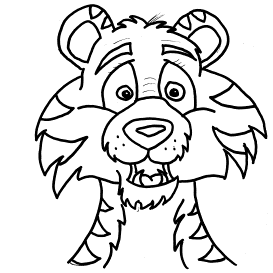1 4 + 0 1 9 + 6 1 0 + 3 1 8 + 1 1 4 + 5 1 4 + 9 1 1 + 3 1 7 + 4Subtraction 3

 8    - 6 5    - 12    - 1 10    - 6 8    - 0 8    - 2 0    - 0 2    - 0 3    - 0 3    - 15    + 3 0    + 7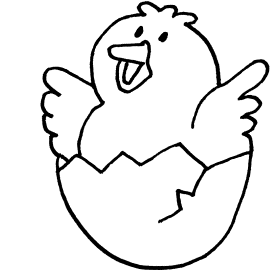7    + 3 7    + 0 10    + 3 9    + 9 9    + 3 0    + 3 8    + 6 1    + 7Missing Numbers 2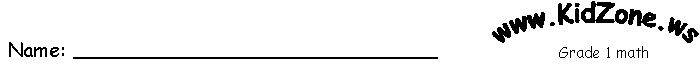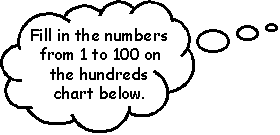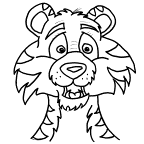____ 2 3 4 ____ ____ ____ ____ ____ ____ ____ ____ ____ ____ 15 ____ ____ ____ 19 20 21 ____ 23 ____ ____ ____ ____ ____ ____ 30 ____ ____ 33 ____ ____ 36 ____ 38 ____ ____ ____ 42 ____ ____ ____ ____ ____ 48 49 ____ ____ 52 53 ____ ____ ____ 57 ____ ____ ____ ____ ____ ____ ____ ____ 66 ____ ____ 69 70 ____ ____ 73 ____ ____ ____ ____ ____ 79 80 ____ ____ ____ ____ 85 ____ 87 88 ____ ____ ____ ____ ____ ____ ____ 96 97 ____ 99 ___

Missing Numbers 1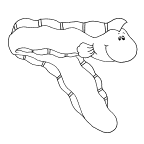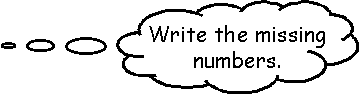31 __ __ __ __ 36 __ __ 39 __

Write the numbers before and after the ones in the large (center) starfish.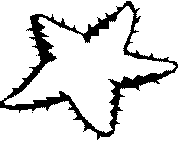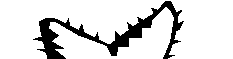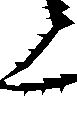38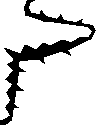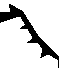35